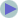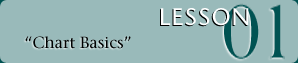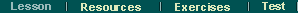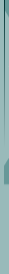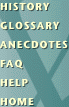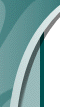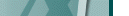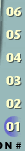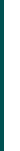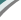Welcome: Lesson 1: Part 1. Attributes of a Chart

Here is a Point and Figure Trend Chart with the characteristics pointed out and described. We will go into detail as we continue through the first lesson.

A Point and Figure Trend Chart:
The trend chart depicts the price action of the stock. We call it the trend chart because of the support and resistance lines that determine whether a chart is above or below trend. If someone say's "...the trend chart" you know they mean this type of chart, this is different from an RS chart for example.

 Key Points: * X's mean the chart is rising * O's mean the chart is declining. * Never will you see X's in a column of O's or vice versa. * Each column must have at least three X's or three O's.

 You will notice some columns have numbers or letters in place of an X or an O. (In blue). These depict months: 1 = January      2 = February     3 = March This continues until October in which... A = October      B = November C = December This is because two digit numbers wouldn't fit in the box.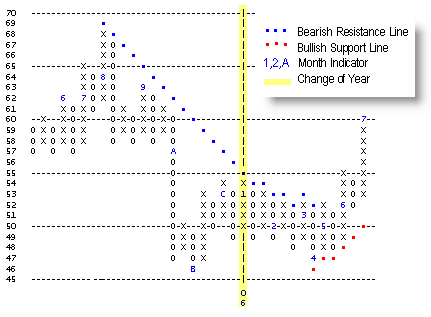Value / Price column The vertical axis is the price scale. From this you can determine the value of each row. Trend Lines: The Bullish Support Lines and Bearish Resistance Lines help us determine the trend of the stock.Next